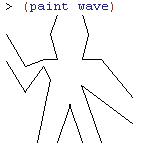## Sunday, October 9, 2011

### SICP 2.49: Defining Primitive Painters

From SICP section 2.2.4 Example: A Picture Language

Exercise 2.49 asks us to use segments->painter to define the following primitive painters:

1. The painter that draws the outline of the designated frame.
2. The painter that draws an "X'' by connecting opposite corners of the frame.
3. The painter that draws a diamond shape by connecting the midpoints of the sides of the frame.
4. The wave painter.

Just as we did in exercises 2.44 & 2.45, we can use the PLT Scheme SICP Picture Language package to run the exercises. You can load the picture package by putting the following (require...) expression at the beginning of your Scheme file.
(require (planet "sicp.ss" ("soegaard" "sicp.plt" 2 1)))

Since segments->painter takes a list of segments as its input parameter, each solution is just a matter of figuring out the coordinates of the endpoints of each required segment. Recall that the (0.0, 0.0) coordinate is the bottom left corner in the frame, not the top left corner as it is in many GUI environments. For each solution, we can define the list of segments first, then define a painter.
; 2.49 a.; The painter that draws the outline of the designated frame.(define outline-segments (list  (make-segment   (make-vect 0.0 0.0)   (make-vect 0.0 0.99))  (make-segment   (make-vect 0.0 0.0)   (make-vect 0.99 0.0))  (make-segment   (make-vect 0.99 0.0)   (make-vect 0.99 0.99))  (make-segment   (make-vect 0.0 0.99)   (make-vect 0.99 0.99))))(define outline (segments->painter outline-segments))

Note that I used 0.99 instead of 1.0 for the edges of the frame. This is because the top and right edges won't be displayed if you use 1.0.; 2.49 b.; The painter that draws an X'' by connecting opposite corners of the frame.(define x-segments (list  (make-segment   (make-vect 0.0 0.0)   (make-vect 0.99 0.99))  (make-segment   (make-vect 0.0 0.99)   (make-vect 0.99 0.0))))(define x-painter (segments->painter x-segments)); 2.49 c.; The painter that draws a diamond shape by connecting the midpoints of the sides of the frame.(define diamond-segments (list  (make-segment   (make-vect 0.0 0.5)   (make-vect 0.5 0.0))  (make-segment   (make-vect 0.0 0.5)   (make-vect 0.5 0.999))  (make-segment   (make-vect 0.5 0.999)   (make-vect 0.999 0.5))  (make-segment   (make-vect 0.999 0.5)   (make-vect 0.5 0.0))))(define diamond (segments->painter diamond-segments))The wave painter in the last part of the exercise is the image shown in Figure 2.10 of the text. It consists of 17 separate segments.
; 2.49 d.; The wave painter.(define wave-segments (list  (make-segment   (make-vect 0.006 0.840)   (make-vect 0.155 0.591))  (make-segment   (make-vect 0.006 0.635)   (make-vect 0.155 0.392))  (make-segment   (make-vect 0.304 0.646)   (make-vect 0.155 0.591))  (make-segment   (make-vect 0.298 0.591)   (make-vect 0.155 0.392))  (make-segment   (make-vect 0.304 0.646)   (make-vect 0.403 0.646))  (make-segment   (make-vect 0.298 0.591)   (make-vect 0.354 0.492))  (make-segment   (make-vect 0.403 0.646)   (make-vect 0.348 0.845))  (make-segment   (make-vect 0.354 0.492)   (make-vect 0.249 0.000))  (make-segment   (make-vect 0.403 0.000)   (make-vect 0.502 0.293))  (make-segment   (make-vect 0.502 0.293)   (make-vect 0.602 0.000))  (make-segment   (make-vect 0.348 0.845)   (make-vect 0.403 0.999))  (make-segment   (make-vect 0.602 0.999)   (make-vect 0.652 0.845))  (make-segment   (make-vect 0.652 0.845)   (make-vect 0.602 0.646))  (make-segment   (make-vect 0.602 0.646)   (make-vect 0.751 0.646))  (make-segment   (make-vect 0.751 0.646)   (make-vect 0.999 0.343))  (make-segment   (make-vect 0.751 0.000)   (make-vect 0.597 0.442))  (make-segment   (make-vect 0.597 0.442)   (make-vect 0.999 0.144))))(define wave (segments->painter wave-segments))Related:

For links to all of the SICP lecture notes and exercises that I've done so far, see The SICP Challenge.